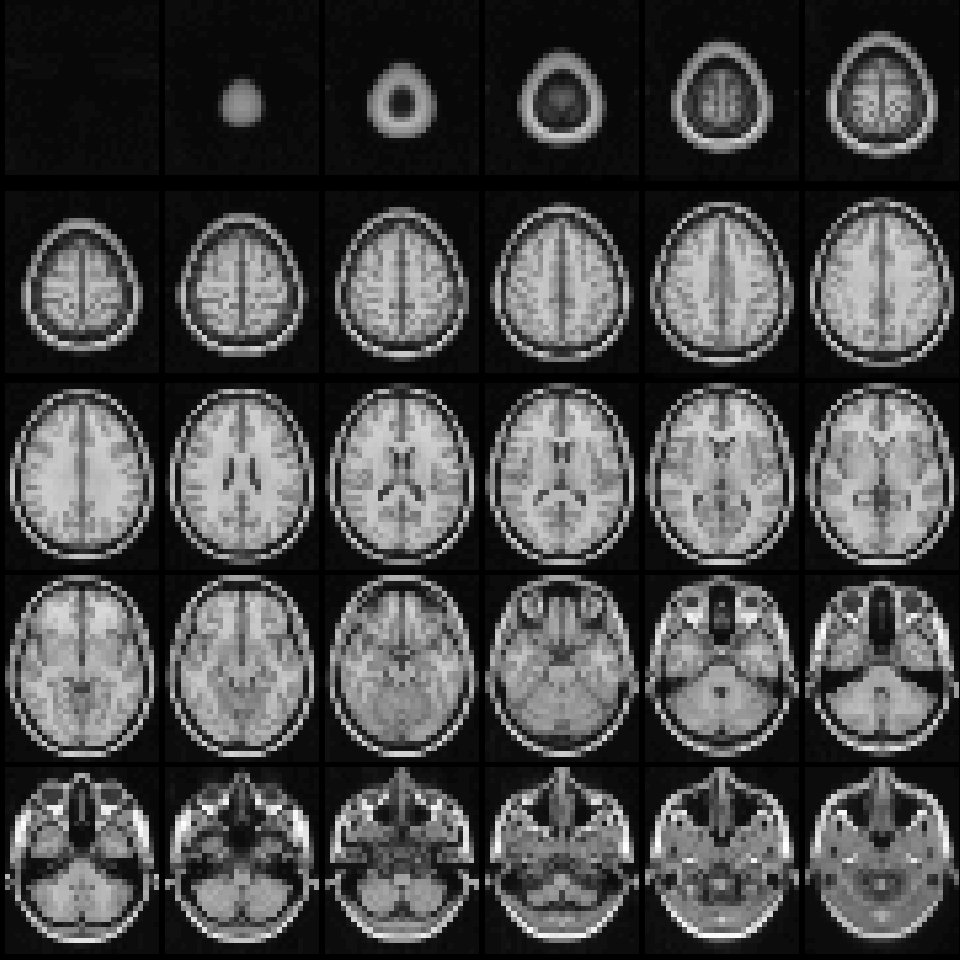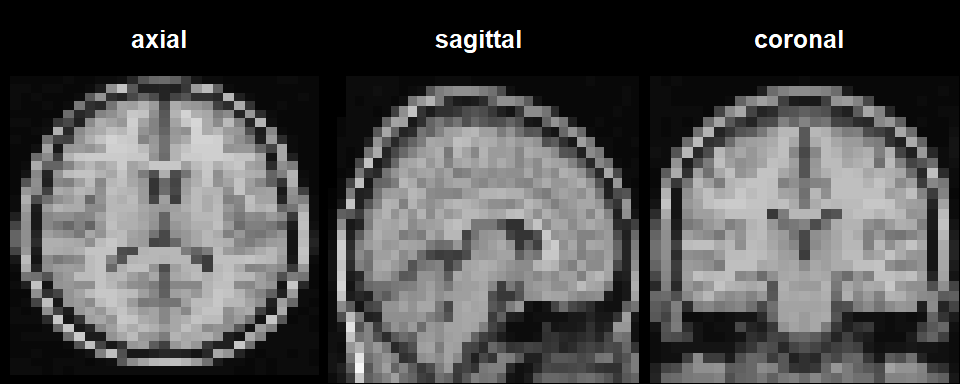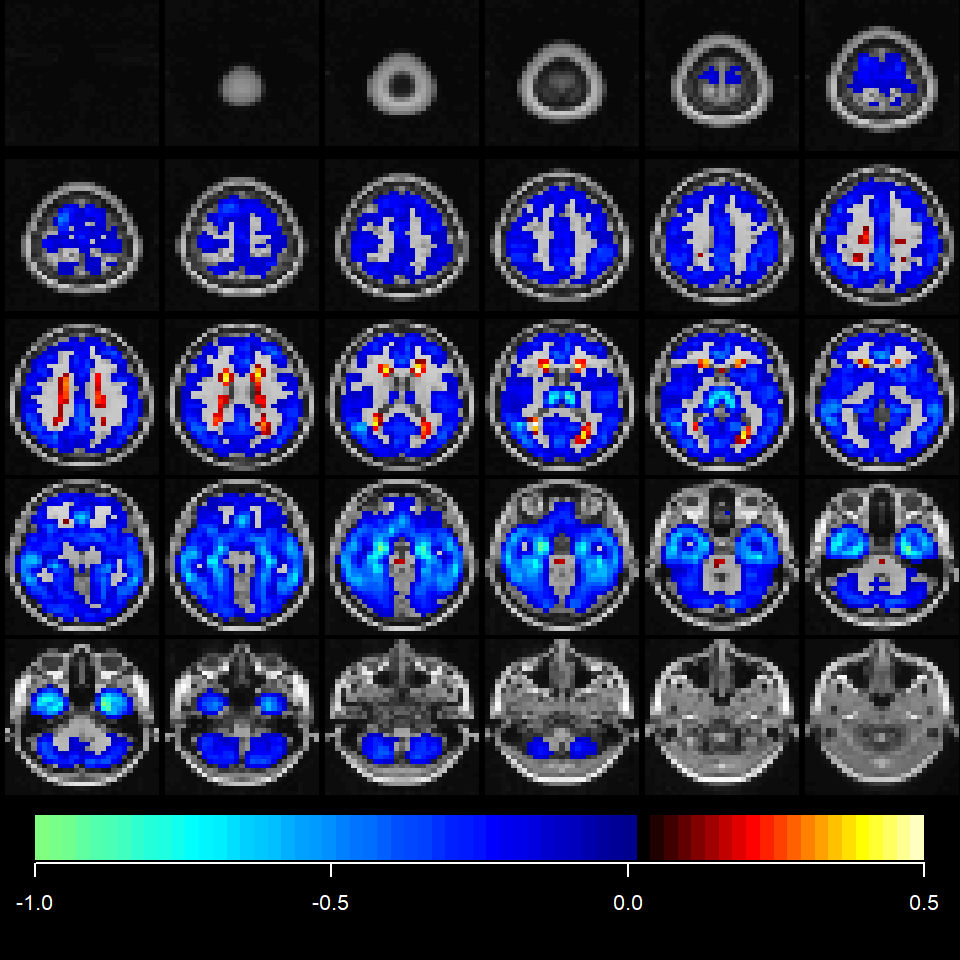2021-06-29 @Atsushi Kawaguchi

The `mand` package provides functions for multivariate analysis for neuroimaging data.

``library(mand)``
``##  要求されたパッケージ msma をロード中です``

# Introduction

## Template

One subject image as the template is available in the mand package. The coad to load it is as follows.

``data(template)``

The image is compressed because of storage and computation time. The dimension is confirmed as follows.

``dim(template)``
``##  30 36 30``

The image is plotted by the `coat` function.

``coat(template)``Other options with the plane argument (such as “axial,” “coronal,” “sagittal,” and “all”) are available. The default setting is “axial”. If the argument is specified as `plane="all"`, three directional slices at a certain coordinate are plotted.

``coat(x=template, plane="all")``## Image Data Matrix

The `imgdatamat` function reads image files saved in the nifti format and creates data matrix with subjects in row and voxel in column (this example does not work).

``````fnames1 = c("data1.nii", "data2.nii")
imgmat = imgdatamat(fnames1, simscale=1/4)``````

The first argument is file names with the length equaling the number of subjects (the number of rows in the resulting data matrix). The second argument `simscale` is the image resize scale. In this example, the all sizes (number of voxel) for three direction was reduced into its quarter size. The output is the list form where the “S” is data matrix, the “brainpos” is a binary image indicating brain region, and the “imagedim” is image dimension. The ROI (Region Of Interest) volume is computed in the “roi” if the ROI argument is TRUE.

``imgmat = imgdatamat(fnames1, simscale=1/4, ROI=TRUE)``

## Overlay

The resulting map from the statistical analysis such as the t statistics map from the SPM is represented with overlapping on the template. For example, the `mand` package has the average difference assuming Alzheimer’s disease and healthy subjects with the array format.

The overlay is implemented by the `coat` function.

``````data(diffimg)
coat(template, diffimg)``````## Atlas

Anatomical brain segmentation region is useful for the plot and the interpretation. For example, the Automated Anatomical Labeling (AAL) atlas is used. The data.frame has two columns (“ROIid” and “ROIname”) format.

``````data(atlasdatasets)
atlasname = "aal"
atlasdataset = atlasdatasets[[atlasname]]
``````##   ROIid                       ROIname
## 0     0                    Background
## 1     1               Left Precentral
## 2     2              Right Precentral
## 3     3         Left Superior Frontal
## 4     4        Right Superior Frontal
## 5     5 Left Superior Frontal Orbital``````

It is also neccesary to prepare the array data.

``````data(atlas)
tmpatlas = atlas[[atlasname]]
dim(tmpatlas)``````
``##  30 36 30``

It has the ROIid as the element.

``tmpatlas[11:15,11:15,10]``
``````##      [,1] [,2] [,3] [,4] [,5]
## [1,]   56   56   56   56   56
## [2,]   98   98   98   56   56
## [3,]   98   98   96   96   96
## [4,]   98   98   96   96   94
## [5,]   98   96   96   96  110``````

The anatomical region can be plotted by the `coat` function with regionplot=TRUE.

``````coat(template, tmpatlas, regionplot=TRUE,
atlasdataset=atlasdataset, ROIids = c(1:2, 37:40),
regionlegend=TRUE)``````